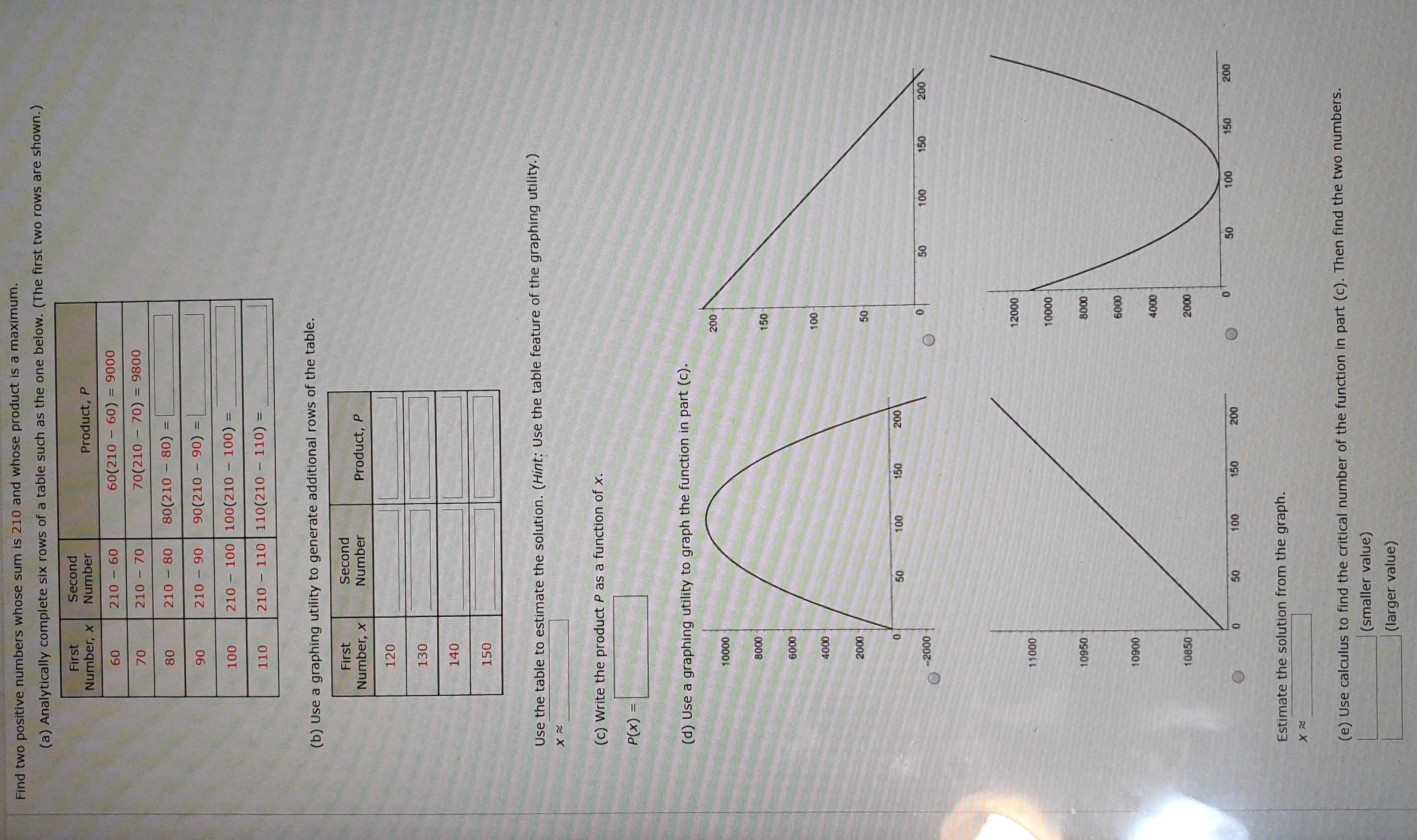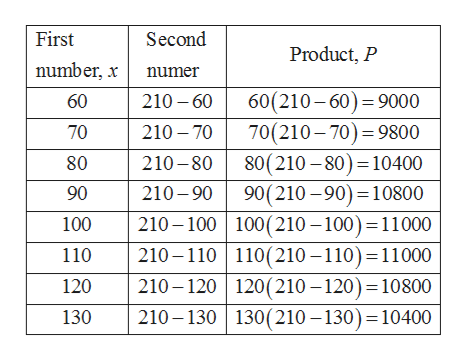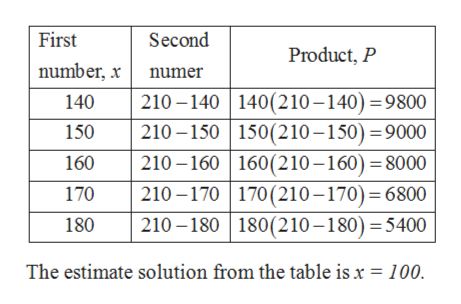150505050505050Find two positive numbers whose sum is 210 and whose product is a maximum.(a) Analytically complete six rows of a table such as the one below. (The first two rows are shown.)FirstNumber, xSecondNumberProduct, P21060(210 60)0000060921070(210 70) = 98000400210080(210 80)210 9006(06 OI)06100210100 100(210 100)110210 110 110(210 110) =(b) Use a graphing utility to generate additional rows of the table.SecondNumberFirstNumber, xProduct, P120130140150Use the table to estimate the solution. (Hint: Use the table feature of the graphing utility.)(c) Write the product P as a function of x(x)d(d) Use a graphing utility to graph the function in part (c).2000000L000150000900L0002000000000-2000000201200011000000010950000109000009000010850000100150200150000000Estimate the solution from the graph.NX(e) Use calculus to find the critical number of the function in part (c). Then find the two numbers.(smaller value)(larger value)

Question

How can I get the result?

Which is the resulthelp_outlineImage Transcriptionclose150 50 50 50 50 50 50 Find two positive numbers whose sum is 210 and whose product is a maximum. (a) Analytically complete six rows of a table such as the one below. (The first two rows are shown.) First Number, x Second Number Product, P 210 60(210 60) 00 0006 09 210 70(210 70) = 9800 04 00 210 0 80(210 80) 210 90 06 (06 OI)06 100 210 100 100(210 100) 110 210 110 110(210 110) = (b) Use a graphing utility to generate additional rows of the table. Second Number First Number, x Product, P 120 130 140 150 Use the table to estimate the solution. (Hint: Use the table feature of the graphing utility.) (c) Write the product P as a function of x (x)d (d) Use a graphing utility to graph the function in part (c). 200 0000L 000 150 0009 00L 000 2000 0 000 00 -2000 00 020 12000 11000 0000 10950 000 10900 0009 0000 10850 000 100 150 200 150 000 00 0 Estimate the solution from the graph. NX (e) Use calculus to find the critical number of the function in part (c). Then find the two numbers. (smaller value) (larger value) fullscreen
Step 1

To find two positive numbers whose sum is 210 and whose product is a maximum.

(a) Analytically complete six rows of the given table.help_outlineImage TranscriptioncloseFirst Second Product, P number, xnumer 60(210-60)9000 70(210 70) 9800 80(210 80) 10400 90(210-90) 10800 210 100 100(210 -100) =11000 210-110 110(210-110) 11000 210-120 120(210 -120) 10800 210-130 130(210 -130) 10400 60 210 60 70 210 70 80 210-80 210-90 90 100 110 120 130 fullscreen
Step 2

(b) Use a graphing utility to add the rows and fill it.help_outlineImage TranscriptioncloseFirst Second Product, P number, x numer 210-140 140(2 10 -140) 9800 210-150 150(210-150) 9000 210 -160 160(210-160) 8000 210-170 170(210-170) 6800 210 180 180(210-180) 5400 140 150 160 170 180 The estimate solution from the table is x = 100. fullscreen
Step 3

(c) To write the product P as a...

Want to see the full answer?

See Solution

Want to see this answer and more?

Our solutions are written by experts, many with advanced degrees, and available 24/7

See Solution
Tagged in

Functions